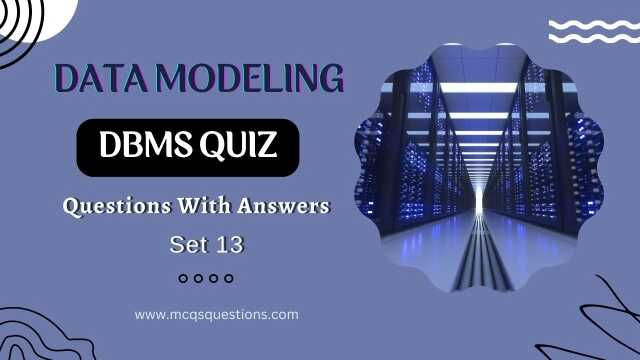# Database Quiz | DBMS Quiz Questions With Answers Set 13

Welcome to our Database Quiz! This quiz is designed to test your knowledge of database management systems (DBMS) and data modeling. Whether you're a beginner or an expert, this quiz will provide you with a fun and interactive way to learn and practice your skills.

This trivia db quiz will cover a wide range of data modeling topics. The quiz questions are multiple-choice, and the answers are provided at the end of each quiz question. We enlist 30 database quiz questions in this DBMS quiz set 13. So, take a moment to brush up on your knowledge, and let's get started!

## Database Management Quiz Set 13

1. What is the process of creating a visual representation of data relationships called?
(A) Data mining
(B) Data modeling
(C) Data warehousing
(D) Data governance

2. What type of data model represents data in a hierarchical tree-like structure?
(A) Object-oriented model
(B) Relational model
(C) Network model
(D) Hierarchical model

3. What is the process of breaking down a large table into smaller, more manageable tables called?
(A) Denormalization
(B) Normalization
(C) Indexing
(D) Partitioning

4. What type of data model represents data in a graph-like structure?
(A) Relational model
(B) Hierarchical model
(C) Object-oriented model
(D) Network model

5. What is the process of creating a simplified version of a complex data model called?
(A) Partitioning
(B) Denormalization
(C) Simplification
(D) Normalization

6. What type of data model represents data in a tabular format?
(A) Object-oriented model
(B) Relational model
(C) Hierarchical model
(D) Network model

7. What is the process of creating a detailed version of a simple data model called?
(A) Normalization
(B) Simplification
(C) Denormalization
(D) Partitioning

8. What type of data model represents data in a document format?
(A) Relational model
(B) Hierarchical model
(C) Document model
(D) Object-oriented model

9. What is the process of creating a logical representation of data called?
(A) Physical modeling
(B) Logical modeling
(C) Conceptual modeling
(D) Data warehousing

10. What is the process of creating a physical representation of data called?
(A) Data warehousing
(B) Logical modeling
(C) Physical modeling
(D) Conceptual modeling

11. What is the process of creating a conceptual representation of data called?
(A) Conceptual modeling
(B) Logical modeling
(C) Physical modeling
(D) Data warehousing

12. What is the process of creating a prototype of a data model called?
(A) Logical modeling
(B) Conceptual modeling
(C) Physical modeling
(D) Prototyping

13. What type of data model represents data in a flexible format?
(A) Relational model
(B) Hierarchical model
(C) Object-oriented model
(D) Document model

14. What is the process of creating a data model that represents the real-world entities and their relationships called?
(A) Entity-Relationship modeling
(B) Logical modeling
(C) Physical modeling
(D) Conceptual modeling

15. What is the process of creating a data model that represents the business rules and constraints called?
(A) Physical modeling
(C) Conceptual modeling
(D) Logical modeling

16. What is the process of creating a data model that represents the data storage and access methods called?
(A) Conceptual modeling
(B) Logical modeling
(C) Data access modeling
(D) Physical modeling

17. What is the process of creating a data model that represents the performance and scalability requirements called?
(A) Performance modeling
(B) Conceptual modeling
(C) Logical modeling
(D) Physical modeling

18. What is the process of creating a data model that represents the security and compliance requirements called?
(A) Conceptual modeling
(B) Logical modeling
(C) Physical modeling
(D) Security modeling

19. What is the process of creating a data model that represents the integration and replication requirements called?
(A) Conceptual modeling
(B) Physical modeling
(C) Integration modeling
(D) Logical modeling

20. What is the process of creating a data model that represents the data lineage and traceability requirements called?
(A) Conceptual modeling
(B) Logical modeling
(C) Data lineage modeling
(D) Physical modeling

21. What is the process of creating a data model that represents the data relationships and constraints called?
(A) Conceptual modeling
(B) Logical modeling
(C) Physical modeling
(D) Data constraint modeling

22. What is the process of creating a data model that represents the data flow and transformation called?
(A) Data flow modeling
(B) Data transformation modeling
(C) Data integration modeling
(D) Data lineage modeling

23. What is the process of creating a data model that represents the data quality and validation rules called?
(A) Data rule modeling
(B) Data validation modeling
(C) Data governance modeling
(D) Data quality modeling

24. What is the process of creating a data model that represents the data lineage and traceability called?
(A) Data quality modeling
(B) Data lineage modeling
(C) Data governance modeling
(D) Data traceability modeling

25. What is the process of creating a data model that represents the data security and access controls called?
(A) Data security modeling
(B) Data governance modeling
(C) Data access control modeling
(D) Data quality modeling

26. What is the process of creating a data model that represents the data archiving and retention policies called?
(A) Data retention modeling
(B) Data quality modeling
(C) Data governance modeling
(D) Data archiving modeling

27. What is the process of creating a data model that represents the data integration and replication requirements called?
(A) Data governance modeling
(B) Data quality modeling
(C) Data replication modeling
(D) Data integration modeling

28. What are the different types of data modeling?
(A) Conceptual, Logical and Physical
(B) Logical, Physical, and Conceptual
(C) Physical, Conceptual, and Logical
(D) Conceptual, Physical, and Logical

29. How do you ensure data integrity in data modeling?
(A) By using data validation rules
(B) By using data constraints
(C) By normalizing data
(D) All of the above

30. What is the process of creating a data model that represents the data backup and disaster recovery requirements called?
(A) Data governance modeling
(B) Data disaster recovery modeling
(C) Data backup modeling
(D) Data quality modeling